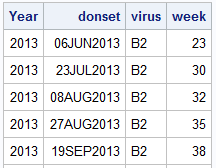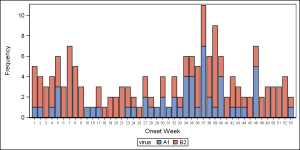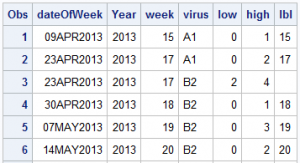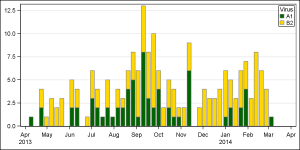Recently, a user posed a question on how to plot stacked frequencies on a time axis.  The data included frequencies of different viruses by week.  The data is modified to preserve confidentiality and is shown below.The user's first instinct was to use a bar chart with stacked groups.  This works for automatically computing frequencies by week and group and also stacked the group values.  Except, the x axis is made discrete and the bars are only drawn where data exists.  However, the user wants to see all weeks positioned correctly the x axis, with gaps where there is no data for some weeks.  The data starts in April 2013 and goes to March 2014, so plotting by week displays the data out of order.

Here is the graph, created using the bar chart.  The graph shows the frequencies for the two viruses by week, using stacked groups.  The data for week numbers 1-14 are listed first even though these actually for 2014.  The weeks are drawn as discrete values, and there are no gaps for weeks that are missing because the bar chart treats the Category axis as discrete.  However, the VBAR statement makes it easy to see the stacked frequencies.To get this kind of graph on a scaled time axis, one would need to use a Needle plot or a HighLow plot.  However, neither of these will automatically compute the frequencies by date and group for a stacked display.So, I used the MEANS procedure to compute the frequencies by week and virus.  Then, I ran a data step by year and week to compute the low and high values for each virus in a given week.  I also compute a "date" value for each week of the year.  Here is the data set:

Now, I use the HighLow plot to draw the bar segments for each virus value by date.  The low and high values for each group segment are already computed.```proc sgplot data=stacked dattrmap=attrmap; format week 2.; highlow x=dateOfWeek low=low high=high / group=virus name='a' type=bar lineattrs=graphdatadefault attrid=virus; yaxis display=(nolabel) offsetmin=0 grid; xaxis display=(nolabel); keylegend 'a' / title='Virus' location=inside position=topright across=1; run;```

As you can see, the SGPLOT code is very simple:

• We use a HighLow plot by dateOfWeek and GROUP=VIRUS.
• We used the previously defined discrete attributes map for each virus name.
• We set other details like legend and axis properties.

The user wanted to see the week values displayed, which can be easily done using the LOWLABEL option of the HighLow plot.

The full SAS code is snown below, however, I cannot share the data as it is confidential.  You can see the structure of the data above and if you simulate similar data, you can run the code.

Full SAS 9.3 program (not including data): HighLow_Timeline

ShareDirector, R&D

Sanjay Matange is R&D Director in the Data Visualization Division responsible for the development and support of the ODS Graphics system, including the Graph Template Language (GTL), Statistical Graphics (SG) procedures, ODS Graphics Designer and related software. Sanjay has co-authored a book on SG Procedures with SAS/PRESS.

### Related Posts

1.•